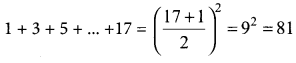## Tamilnadu Samacheer Kalvi 11th Maths Solutions Chapter 4 Combinatorics and Mathematical Induction Ex 4.5

Question 1.
The sum of the digits at the 10th place of all numbers formed with the help of 2, 4, 5, 7 taken all at a time is ……..
(a) 432
(b) 108
(c) 36
(d) 18
Solution:
(b) 108
Hint. Number of digits given = 2, 4, 5, 7
Number of 4 digit numbers formed = 4! =24
So each digit occur $$\frac{24}{4}$$ = 6 times
Sum of the digits = 2 + 4 + 5 + 7 = 18
So sum of the digits in each place = 18 × 6 = 108

Question 2.
In an examination there are three multiple choice questions and each question has 5 choices. Number of ways in which a student can fail to get all answer correct is ……..
(a) 125
(b) 124
(c) 64
(d) 63
Solution:
(b) 124
Hint. Each question has 5 options in which 1 is correct
So the number of ways of getting correct answer for all the three questions is 53 = 125
So the number of ways in which a student can fail to get all answer correct is < 125 (i.e.) 125 – 1 = 124Question 3.
The number of ways in which the following prize be given to a class of 30 boys first and second in mathematics, first and second in physics, first in chemistry and first in English is ……….
(a) 304 × 292
(b) 303 × 293
(c) 302 × 294
(d) 30 × 295
Solution:
(a) 304 × 292
Hint.
I and II in maths can be given can be given in 30 × 29 ways.
I and II in physics can be given in 30 × 29 ways.
I and chemistry can be given in 30 ways.
I in English can be given in 30 ways.
So total number of ways = 30 × 29 × 30 × 29 × 30 × 30 = 304 × 292Question 4.
The number of 5 digit numbers all digits of which are odd is ………
(a) 25
(b) 55
(c) 56
(d) 625
Solution:
(b) 55
Hint. The odd number are 1, 3, 5, 7, 9
Number of odd numbers = 5
We need a five digit number So the number of five digit number = 55

Question 5.
In 3 fingers, the number of ways four rings can be worn is …… ways.
(a) 43 – 1
(b) 34
(c) 68
(d) 64
Solution:
(b) 34
Hint. Each letter can be ported in 3 ways
∴ 4 letter is 34 ways

Question 6.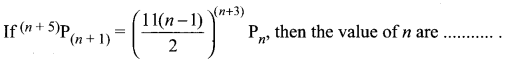(a) 7 and 11
(b) 6 and 7
(c) 2 and 11
(d) 2 and 6
Solution:
(b) 6 and 7

Question 7.
The product of r consecutive positive integers is divisible by ………
(a) r!
(b) (r – 1)!
(c) (r + 1)!
(d) r!
Solution:
(a) r!
Hint.
1(2) (3) ….. (r) = r! which is ÷ by r!

Question 8.
The number of 5 digit telephone numbers which have none of their digits repeated is
(a) 90000
(b) 10000
(c) 30240
(d) 69760
Solution:
(d) 69760
Hint.
The number of 5 digit telephone numbers which have none of their digits repeated is 10P5 = 30240
Thus the required number of telephone number is 105 – 30240 = 69760Question 9.
If a2aC2 = a2aC4 then the value of ‘a’ is ….
(a) 2
(b) 3
(c) 4
(d) 5
Solution:
(b) 3
Hint.
a2 – a = 2 + 4 = 6
a2 – a – 6 = 0
(a – 3) (a + 2) = 0 ⇒ a = 3

Question 10.
There are 10 points in a plane and 4 of them are collinear. The number of straight lines joining any two points is ……..
(a) 45
(b) 40
(c) 39
(d) 38
Solution:
(b) 40
Hint.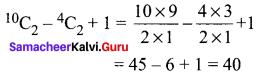Question 11.
The number of ways in which a host lady invite 8 people for a party of 8 out of 12 people of whom two do not want to attend the party together is
(a) 2 × 11C7 + 10C8
(b) 11C7 + 10C8
(c) 12C810C6
(d) 10C6 + 2!
Solution:
(c) 12C810C6
Hint.
Number of way of selecting 8 people from 12 in 12C8
∴ out of remaining people 8 can attend in 10C8
The number of ways in which two of them do not attend together = 12C810C6

Question 12.
The number of parallelograms that can be formed from a set of four parallel lines intersecting another set of three parallel lines …….
(a) 6
(b) 9
(c) 12
(d) 18
Solution:
(d) 18
Hint.
Number of parallelograms = 4C2 × 3C2
= 6 × 3 = 18Question 13.
Everybody in a room shakes hands with everybody else. The total number of shake hands is 66. The number of persons in the room is …….
(a) 11
(b) 12
(c) 10
(d) 6
Solution:
(b) 12
Hint.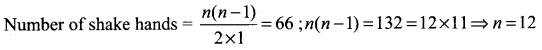Question 14.
Number of sides of a polygon having 44 diagonals is ……….
(a) 4
(b) 4!
(c) 11
(d) 22
Solution:
(c) 11
Hint: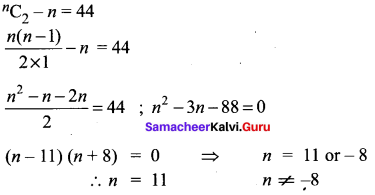Question 15.
If 10 lines are drawn in a plane such that no two of them are parallel and no three are concurrent, then the total number of points of intersection are ………
(a) 45
(b) 40
(c) 10!
(d) 210
Solution:
(a) 45
Hint: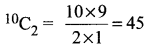Question 16.
In a plane there are 10 points are there out of which 4 points are collinear, then the number of triangles formed is …….
(a) 110
(b) 10C3
(c) 120
(d) 116
Solution:
(d) 116
Hint: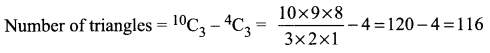Question 17.
In 2nC3 : nC3 = 11 : 1 then n is ………
(a) 5
(b) 6
(c) 11
(d) 1
Solution:
(b) 6
Hint.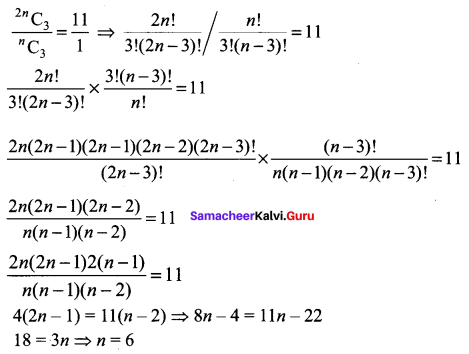Question 18.
(n – 1)Cr + (n – 1)C(r – 1) is ………
(a) (n + 1)Cr
(b) (n – 1)Cr
(c) nCr
(d) nCr – 1
Solution:
(c) nCr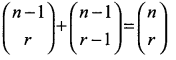Question 19.
The number of ways of choosing 5 cards out of a deck of 52 cards which include at least one king is …….
(a) 52C5
(b) 48C5
(c) 52C5 + 48C5
(d) 52C548C5
Solution:
(d) 52C548C5
Hint.
Selecting 5 from 52 cards = 52C5
selecting 5 from the (non-king cards 48) = 48C5
∴ Number of ways is 52C548C5

Question 20.
The number of rectangles that a chessboard has ……
(a) 81
(b) 99
(c) 1296
(d) 6561
Solution:
(c) 1296
Hint. Number of horizontal times = 9
Number of vertical times = 9
Selecting 2 from 9 horizontal lines = 9C2
Selecting 2 from 9 vertical lines = 9C2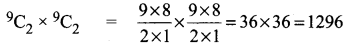Question 21.
The number of 10 digit number that can be written by using the digits 2 and 3 is ……..
(a) 10C2 + 9C2
(b) 210
(c) 210 – 2
(d) 10!
Solution:
(b) 210
Hint.
Selecting the number from (2 and 3)
For till the first digit can be done in 2 ways
For till the second digit can be done in 2 ways ….
For till the tenth digit can be done in 2 ways
So, total number of ways in 10 digit number = 2 × 2 × 2 × 2 × 2 × 2 × 2 × 2 × 2 × 2 = 210Question 22.
If Pr stands for rPr then the sum of the series 1 + P1 + 2P2 + 3P3 + … + nPn is ……..
(a) Pn + 1
(b) Pn + 1 – 1
(c) Pn – 1 + 1
(d) (n + 1)P(n – 1)
Solution:
(b) Pn + 1 – 1
Hint:
1 + 1! + 2! + 3! + … + n!
Now 1 + 1 (1!) = 2 = (1 + 1)!
1 + 1 (1!) + 2(2!) = 1 + 1 + 4 = 6 = 3!
1 + 1(1!) + 2(2!)+ 3(3!) = 1 + 1 + 4 + 18 = 24 = 4!
1 + 1(1!) + 2(2!) + 3(3!) ….+ n(n!) = (n + 1) ! – 1
= n + 1Pn + 1 – 1 = Pn + 1 – 1

Question 23.
The product of first n odd natural numbers equals …….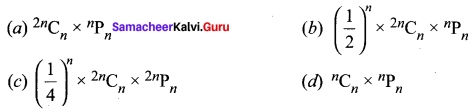Solution: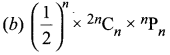Hint: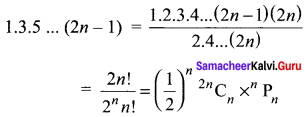Question 24.
If nC4, nC5, nC6 are in AP the value of n can be ………..
(a) 14
(b) 11
(c) 9
(d) 5
Solution:
(a) 14
Hint: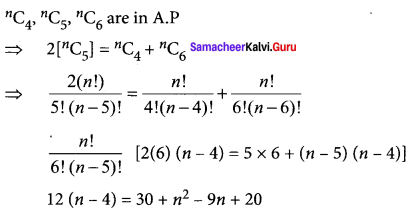30 + n2 – 9n + 20 – 12n + 48 = 0
n2 – 21 n + 98 = 0
(n – 7) (n – 14) = 0
n = 7 (or) 14

Question 25.
1 + 3 + 5 + 7 + + 17 is equal to ………
(a) 101
(b) 81
(c) 71
(d) 61
Solution:
(b) 81
Hint: